New Student User - Use Code HELLO

# Short Tricks to Solve Quadratic Equations## Roots of a Quadratic Equation:

• The values of variable which satisfies the quadratic equation is called solution of the quadratic equation or the roots of the equation.
The roots of quadratic equation is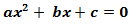is:whereis called discriminant of the quadratic equation and it is represented by D.

## Solution of a Quadratic Equation:

### (i) By Factorisation Method:

if the factors of the given equation  is such that
(dx + e) (fx + g), d≠0, f≠0

### (ii) By Shreedharacharaya formula:

The following formula could be used for equation:If roots of the quadratic equation is α , β , then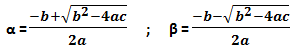## Nature of Roots of Quadratic Equation :

The nature of roots of the quadratic equations are dependent upon the discriminant

D =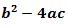, the following table will be helpful to find the nature of roots of a quadratic equation:Also, if roots of the quadratic equationis α, β, then

Sum of roots,and product of roots,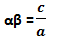## Problem Solving Tricks :

• If α and β are the given roots of the quadratic equation , then you can form the equation as;## Symmetrical Functions of Root :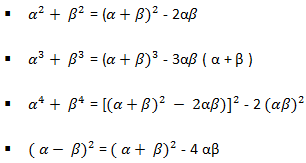Good Luck!